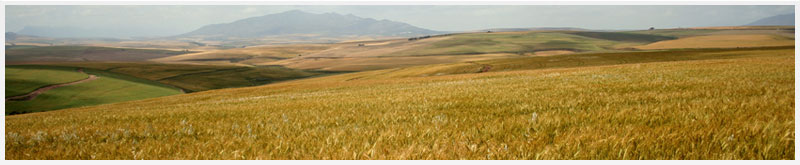## 525 Harvey Street, Halstead, KS, 67056You may sign up to our mailing list below. Only the bolded fields are required. You may unsubscribe here.

####  ####  ##########  ##########    ######    ##########
#::#  #::#  #::####::#  #::#######  ##########  #::#######
#::#  #::#  #::#  #::#  #::#        ##      ##  #::#
#::####::#  #::#  #::#  #::#                ##  #::#
#::::::::#  #::####::#  #::#######       ####   #::#######
####::####  #::####::#  #::####::#       ####   #######::#
#::#     #::#  #::#  #::#  #::#          ##        #::#
#::#     #::#  #::#  #::#  #::#  ##      ##        #::#
#::#     #::####::#  #::####::#  ##########  #######::#
####     ##########  ##########    ######    ##########